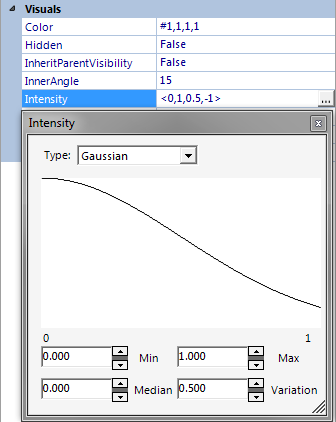# Gaussian

Jump to: navigation, searchA Gaussian curve, allowing for the modification of the intensity of an effect

Gaussian, within the context of HeroEngine, is the name of some math functions, and a term for a graphics technique, using mathematics and probability as developed by Carl Friedrich Gauss. "Gaussian" is one of the options that can be used in many dropdown menus, such as for the intensity of an effect.

## Gaussian parameters on effects

When choosing a Gaussian Parameter syntax, this means to provide a random number within the specified range, with the given median and variation.
• A Gaussian RGB color parameter is an extension of the RGB color parameter type. Any of the elements r, g, b and a may be either a value or a Gaussian. Gaussian parameters are reevaluated every frame, causing a flickering effect useful for torches, fluorescent lights, etc.
• A Gaussian vector parameter has the syntax: (x,y,z), where each element in the vector can be either a float or a Gaussian parameter. These are particularly useful for the Trajectory parameter on particle specs, as the parameter is sampled once per particle when it is emitted.

## Gaussian functions

`Gaussian(min as float, max as float, standardDeviation as float, median as float) as float`

`GaussianDeterministic(min as float, max as float, standardDeviation as float, median as float) as float`

These functions can be used to generate random numbers with a Gaussian distribution, though simpler functions are usually sufficient. See Random number generation.